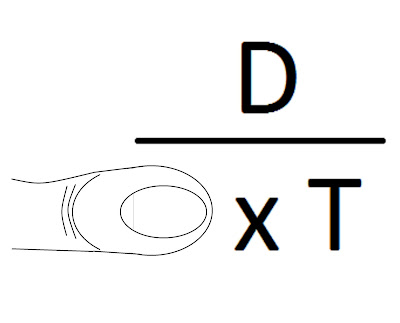## Friday 20 July 2018

### Distance Speed & Time

Speed in Knots-Distance-Time
Knots        Metres    per
Hour            Minute        Second
1        1,852.00        30.866        0.5144
2        3,704.00        61.733        1.0288
3        5,556.00        92.600        1.5432
4        7,408.00        123.467      2.0576
5        9,260.00        154.334      2.5720
6        11,112.00       185.201     3.0864
7        12,964.00       216.068     3.6008
8        14,816.00       246.935     4.1152
9        16,668.00       277.802     4.6296
10      18,520.00       308.669     5.1440

One knot is one nautical mile per hour, two knots is two nautical miles per hour and so forth. The knot is a non-SI unit that is accepted for use with the SI (International Standard). A vessel travelling at 1 knot along a meridian travels approximately one minute of geographic latitude in one hour.

------------------------------------------------------------------------------------------------------------------

Formulas for working Distance Speed and Time

D = S X T
T = D / S
S = D / T

D
-----
S X T

A simple way to remember the formulas is to think of a pyramid or sketch it out on paper with the D over the S multiplied by T as in the above figure. If you cover the letter corresponding to the answer you require you will see the formula.

For example 1 if you require to know Speed:

Place your thumb on the S and you will see that to arrive at the correct answer will require Distance to be divided by Time (D over T).Example 1